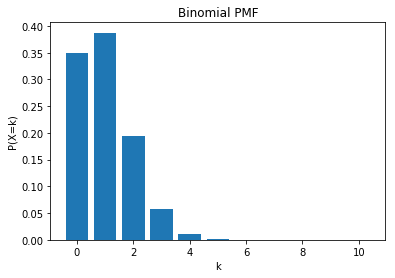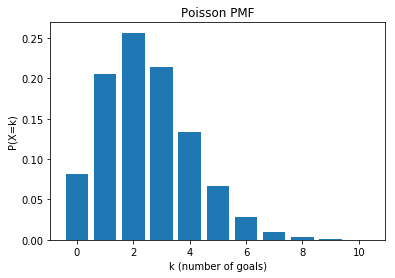# Bernoulli and Binomial Distributions

A Bernoulli Distribution is the probability distribution of a random variable which takes the value 1 with probability p and value 0 with probability 1 – p, i.e.

$$\begin{cases} 1-p & \text{for}\ k=0 \\ p & \text{for}\ k=1 \\ \end{cases}$$

We will use the example of left-handedness. Approximately 10% of the population are left-handed (p=0.1).

We want to know, out of a random sample of 10 people, what is the probability of 3 of these 10 people being left handed?

We assign a 1 to each person if they are left handed and 0 otherwise:

• $P(X=1) = 0.1$
• $P(X=0) = 0.9$

A Binomial distribution is derived from the Bernoulli distribution.

What is the probability of the first 3 people we pick being left-handed, followed by 7 people being right-handed?

This is just $0.1 ^3 \times 0.9 ^7$

In :
0.1 ** 3 * 0.9 ** 7

Out:
0.0004782969000000002

What if we wanted the last 3 people to be left-handed?

This is just $0.9^7 \times 0.1^3$, the same answer.

In fact, no matter how we arrange the 3 people, we will always end up with the same probability ($4.7 \times 10^{-4}$).

So we have to add up all the ways we can arrange the 3 people being picked.

There are $10!$ ways to arrange 10 people and there are $3!$ ways to arrange the 3 people that are picked and $7!$ ways to arrange the 7 people that aren’t picked.

This is given as:
$$\dfrac{10!}{3!\ 7!}$$

In :
from math import factorial

factorial(10) / (factorial(3) * factorial(7))

Out:
120.0

Or more commonly, “10 choose 3”. The “n choose k” notation is written as:
$$\begin{equation*} \binom{n}{k} \end{equation*} = \dfrac{n!}{k!\ (n-k)!}$$

We can now caclulate the probability that there are 3 left-handed people in a random selection of 10 people as:

$$P(X=3) = \begin{equation*} \binom{10}{3} \end{equation*} (0.1)^3 (0.9)^7$$

In :
(factorial(10) / (factorial(3) * factorial(7))) * 0.1 ** 3 * 0.9 ** 7

Out:
0.05739562800000002

$P(X=3) = 0.057$

This will generalise such that:

$$P(X=k) = \begin{equation*} \binom{n}{k} \end{equation*} (p)^k (1-p)^{n-k}$$

Scipy’s stats package has a binomial package that can be used to calculate these probabilities:

In :
# parameters are k, n and p
from scipy.stats import binom

binom.pmf(3, 10, 0.1)

Out:
0.057395628000000067

We can use this function to calculate what the probability of 3 or fewer people being left-handed from a selection of 10 people.

$$P(X \leq 3) = \sum_{i=0}^{3} \begin{equation*} \binom{10}{i} \end{equation*} (0.1)^i (0.9)^{n-i}$$

In :
sum([binom.pmf(x, 10, 0.1) for x in range(4)])

Out:
0.98720480160000057

$P(X \leq 3) = 0.987$

Or we could plot our probability results for each value up to all 10 people being left-handed:

In :
import matplotlib.pyplot as plt

plt.bar(range(11), [binom.pmf(x, 10, 0.1) for x in range(11)])
plt.xlabel('k')
plt.ylabel('P(X=k)')
plt.title('Binomial PMF')
plt.show()We can see there is almost negligible chance of getting more than 6 left-handed people in a random group of 10 people.

### Roulette

On an American roulette wheel there are 38 squares:

• 18 black
• 18 red
• 2 green

We bet on black 10 times in a row, what are the chances of winning more than half of these?

$$P(X \gt 5) = \sum_{i=6}^{10} \begin{equation*} \binom{10}{i} \end{equation*} \bigg(\dfrac{18}{38}\bigg)^i \bigg(1-\dfrac{18}{38}\bigg)^{n-i}$$

In :
p = 18 / 38
sum([binom.pmf(x, 10, p) for x in range(6, 11)])

Out:
0.31412504396776203

$P(X \gt 5) = 0.314$

# Poisson Distribution

A Poisson distribution is a limiting version of the binomial distribution, where $n$ becomes large and $np$ approaches some value $\lambda$, which is the mean value.

The Poisson distribution can be used for the number of events in other specified intervals such as distance, area or volume. Examples that may follow a Poisson include the number of phone calls received by a call center per hour and the number of decay events per second from a radioactive source.

It is calculated as:

$P(k) = e^{-\lambda} \dfrac{\lambda^k}{k!}$

The average number of goals in a World Cup football match is 2.5.

We would like to know the probability of 4 goals in a match.

In :
from math import exp

_lambda = 2.5
k = 4

(exp(-_lambda)) * _lambda ** k / factorial(k)

Out:
0.13360188578108528

Again, scipy has in-built functions for calculating this and we can use this to calculate the probability of any number of goals in a World Cup match.

In :
# parameters are k and lambda
from scipy.stats import poisson

import matplotlib.pyplot as plt

plt.bar(range(11), [poisson.pmf(k, _lambda) for k in range(11)])
plt.xlabel('k (number of goals)')
plt.ylabel('P(X=k)')
plt.title('Poisson PMF')
plt.show()